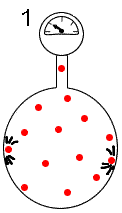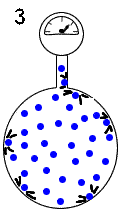# The partial pressure of a gas in a gas mixture

## Qualitative approach

A gas occupies alone a given volume. The pressure gauge measures the pressure resulting from the collisions of the molecules with the wall of the enclosure.A second gas with three times as many molecules occupies now alone the same volume. The pressure gauge measures a pressure which is three time higher as there are three time as many collisions.Now the two gases occupy together the same volume. The number of collisions is equal to the sum of the former number of collisions. So the pressure gauge measures the sum of the former pressures.## Definition of the partial pressure

In a gas mixture, the partial pressure of a gas is the pressure of this gas supposing that it occupies alone the whole volume (at the same temperature).

## Relation between partial pressure and number of moles

Consider a mixture of $n_1$ moles of a gas with $n_2$ moles of a second gas in the volume $V$ at $T$ Kelvin degrees The ideal gas law and the preceding definition allow us to calculate the partial pressures:

$P_1$ $=$ $n_1 \cdot \frac{R\cdot T}{V}$ $P_2$ $=$ $n_2 \cdot \frac{R\cdot T}{V}$

## Relation between partial pressure and molarity

As $\frac{n}{V}$ is the molar concentration (molarity), we have:

$P_1$ $=$ $ \cdot R\cdot T$ $P_2$ $=$ $ \cdot R\cdot T$ where $$ and $$ are the molarities of the two gases

## Relation between partial pressures and the total pressure

The total number of moles of the gas mixture being equal to $n$ $=$ $n_1$ $+$ $n_2$, the total pressure $P$ of the mixture amounts to: $P$ $=$ $(n_1+n_2) \cdot \frac{R\cdot T}{V}$ $=$ $n_1 \cdot \frac{R\cdot T}{V}$ $+$ $n_2 \cdot \frac{R\cdot T}{V}$

If $P$ is the pressure of a gaseous mixture and $P_1$ and $P_2$ are the partial pressures of the constituants, then $P$ $=$ $P_1$ $+$ $P_2$ The total pressure of a gas mixture is equal to the sum the partial pressure of its constituents.

## Définition of the mole fraction

If $n_1$ and $n_2$ are the numbers of moles of the constituants of a mixture, then the ratios $X_1$ $=$ $\frac{n_1}{n_1+n_2}$ $X_2$ $=$ $\frac{n_2}{n_1+n_2}$ are called mole fractions of 1 and 2.

## Relation between partial pressure and mole fraction

$\frac{P_1}{P}$ $=$ $\frac{n_1 RT}{(n_1+n_2)RT}$ $\frac{P_2}{P}$ $=$ $\frac{n_2 RT}{(n_1+n_2)RT}$

$P_1$ $=$ $X_1P$ $P_2$ $=$ $X_2P$ The partial pressure of a gas in a gas mixture is equal to its mole fraction multiplied by the total pressure of the mixture.